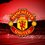# Variants of the Clausen Functions

To Prove: $\sum _{ k=1 }^{ \infty }{ \frac { \cos { \left( kx \right) } }{ { k }^{ 2 } } } =\frac { { \pi }^{ 2 } }{ 6 } -\frac { \pi x }{ 2 } +\frac { x^{ 2 } }{ 4 }$

$0\leq x\leq 2\pi$

Proof:

Write $\cos(kx)$ as: $\displaystyle \cos { \left( kx \right) } =\frac { { e }^{ ikx }+{ e }^{ -ikx } }{ 2 }$

Then we get $S=\sum _{ k=1 }^{ \infty }{ \frac { \frac { { e }^{ ikx }+{ e }^{ -ikx } }{ 2 } }{ { k }^{ 2 } } }$

Then on using the definition of polylogarithm I'll write it as: $S=\frac { { Li }_{ 2 }\left( { e }^{ ikx } \right) +{ Li }_{ 2 }\left( { e }^{ -ikx } \right) }{ 2 }$

Now, I'll use the following relation of polylogarithm and bernoulli numbers: ${ Li }_{ m }(z)\quad =\quad { (-1) }^{ m-1 }{ Li }_{ m }\left( \frac { 1 }{ z } \right) -\frac { { (2\pi i) }^{ m } }{ m! } { B }_{ m }\left( \frac { ln(-z) }{ 2\pi i } +\frac { 1 }{ 2 } \right) /z\notin (0,1)$

Therefore, I'll re-write $S$ as: $S=\frac { 1 }{ 2 } \frac { { \left( 2\pi \right) }^{ 2 } }{ m! } { B }_{ 2 }\left( \frac { x }{ 2\pi } \right) =\frac { { \pi }^{ 2 } }{ 6 } -\frac { \pi x }{ 2 } +\frac { x^{ 2 } }{ 4 }$Note by Aditya Kumar
5 years, 3 months ago

This discussion board is a place to discuss our Daily Challenges and the math and science related to those challenges. Explanations are more than just a solution — they should explain the steps and thinking strategies that you used to obtain the solution. Comments should further the discussion of math and science.

When posting on Brilliant:

• Use the emojis to react to an explanation, whether you're congratulating a job well done , or just really confused .
• Ask specific questions about the challenge or the steps in somebody's explanation. Well-posed questions can add a lot to the discussion, but posting "I don't understand!" doesn't help anyone.
• Try to contribute something new to the discussion, whether it is an extension, generalization or other idea related to the challenge.
• Stay on topic — we're all here to learn more about math and science, not to hear about your favorite get-rich-quick scheme or current world events.

MarkdownAppears as
*italics* or _italics_ italics
**bold** or __bold__ bold
- bulleted- list
• bulleted
• list
1. numbered2. list
1. numbered
2. list
Note: you must add a full line of space before and after lists for them to show up correctly
paragraph 1paragraph 2

paragraph 1

paragraph 2

[example link](https://brilliant.org)example link
> This is a quote
This is a quote
    # I indented these lines
# 4 spaces, and now they show
# up as a code block.

print "hello world"
# I indented these lines
# 4 spaces, and now they show
# up as a code block.

print "hello world"
MathAppears as
Remember to wrap math in $$ ... $$ or $ ... $ to ensure proper formatting.
2 \times 3 $2 \times 3$
2^{34} $2^{34}$
a_{i-1} $a_{i-1}$
\frac{2}{3} $\frac{2}{3}$
\sqrt{2} $\sqrt{2}$
\sum_{i=1}^3 $\sum_{i=1}^3$
\sin \theta $\sin \theta$
\boxed{123} $\boxed{123}$

## Comments

Sort by:

Top Newest

Alternatively, show that the LHS is the Fourier Series of the RHS for $0\leq x\leq 2\pi$. It suffices to show that $\displaystyle \int_{0}^{2\pi}\left(\frac{\pi^2}{6}-\frac{\pi x}{2}+\frac{x^2}{4}\right)\cos(kx)dx=\frac{\pi}{k^2}$, a simple exercise in calculus.

Note that the equation holds for $0\leq x\leq 2\pi$ only, a fact that should be stated in the problem.

- 5 years, 3 months ago

Log in to reply

Wow. Thanks.

- 5 years, 3 months ago

Log in to reply

Sir why did you remove your problem?

- 5 years, 2 months ago

Log in to reply

I had no idea the same problem was posed before... It can happen to all of us

- 5 years, 2 months ago

Log in to reply

Sir can you give me the link?

- 5 years, 2 months ago

Log in to reply

Somebody posted it as a comment to the problem I have deleted... I really don't remember it... it was an old problem that I must have missed

- 5 years, 2 months ago

Log in to reply

Can you at least post your method?

- 5 years, 2 months ago

Log in to reply

I'm at work now, but I can post something in the evening. Again, I was using Fourier series.

- 5 years, 2 months ago

Log in to reply

Sure sir no problem.

- 5 years, 2 months ago

Log in to reply

Maybe you can try it yourself: Write the Fourier series of $x$ and $x^3$ on $[\pi,\pi]$; then take the linear combination that gives you what you want.

- 5 years, 2 months ago

Log in to reply

I'm trying that :)

- 5 years, 2 months ago

Log in to reply

Sir is this your book? I seriously liked it.

- 5 years, 2 months ago

Log in to reply

Yes, I must confess, it is.... the book is generally not very popular with students (but it is used at many of the best universities in the US)

- 5 years, 2 months ago

Log in to reply

There is a sign error: We have $\cos(kx)=\frac{e^{ikx}+e^{-ikx}}{2}$

- 5 years, 3 months ago

Log in to reply

Thanks for mentioning it sir. It was a typo. While doing it on paper I hadn't made that mistake.

- 5 years, 3 months ago

Log in to reply

×

Problem Loading...

Note Loading...

Set Loading...